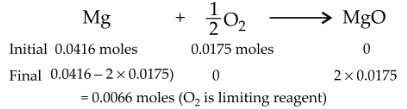Stoichiometry and Stoichiometric Calculations
Question

# 1.0 g of magnesium is burnt with 0.56 g ${\mathrm{O}}_{2}$  in a closed vessel. Which reactant is left in excess and how much?  (At. wt. Mg = 24,O = 16 )

Moderate
Solution

## ${\mathrm{n}}_{\mathrm{Mg}}=\frac{1}{24}=0.0416\mathrm{moles}$the balanced equation isGet Instant Solutions